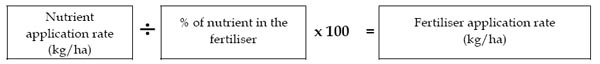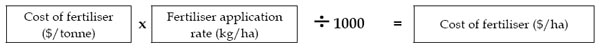To calculate the fertiliser application rate required for a particular
nutrient application rate, use the formula:ReGyp screened gypsum products contain approximately 17% of sulphur (S).
Application Rate x % Sulphur = Sulphur application rate (kg/ha)
Sulphur Kg/ha = 1000 kg/ha x 17 = 170 kg/haReGyp screened gypsum products contain approximately 17% of sulphur (S). Thus, 1 tonne of this gypsum contains 170 kg of sulphur. Using the formula and assuming the cost per tonne of gypsum is \$70 per tonne spread, we can calculate the cost of 1 kg of sulphur in this gypsum.

Cost \$ per tonne / Amount of sulphur in 1 tonne of gypsum = Cost of 1kg of S (\$/kg)
Calculation = \$60/170 = \$0.35/kg of sulphur (S)

You have visited the REGYP News Room, for more info please visit the full REGYP website www.regyp.com.au , or call 1300 4 REGYP (73497) or email info@regyp.com.au for more information.